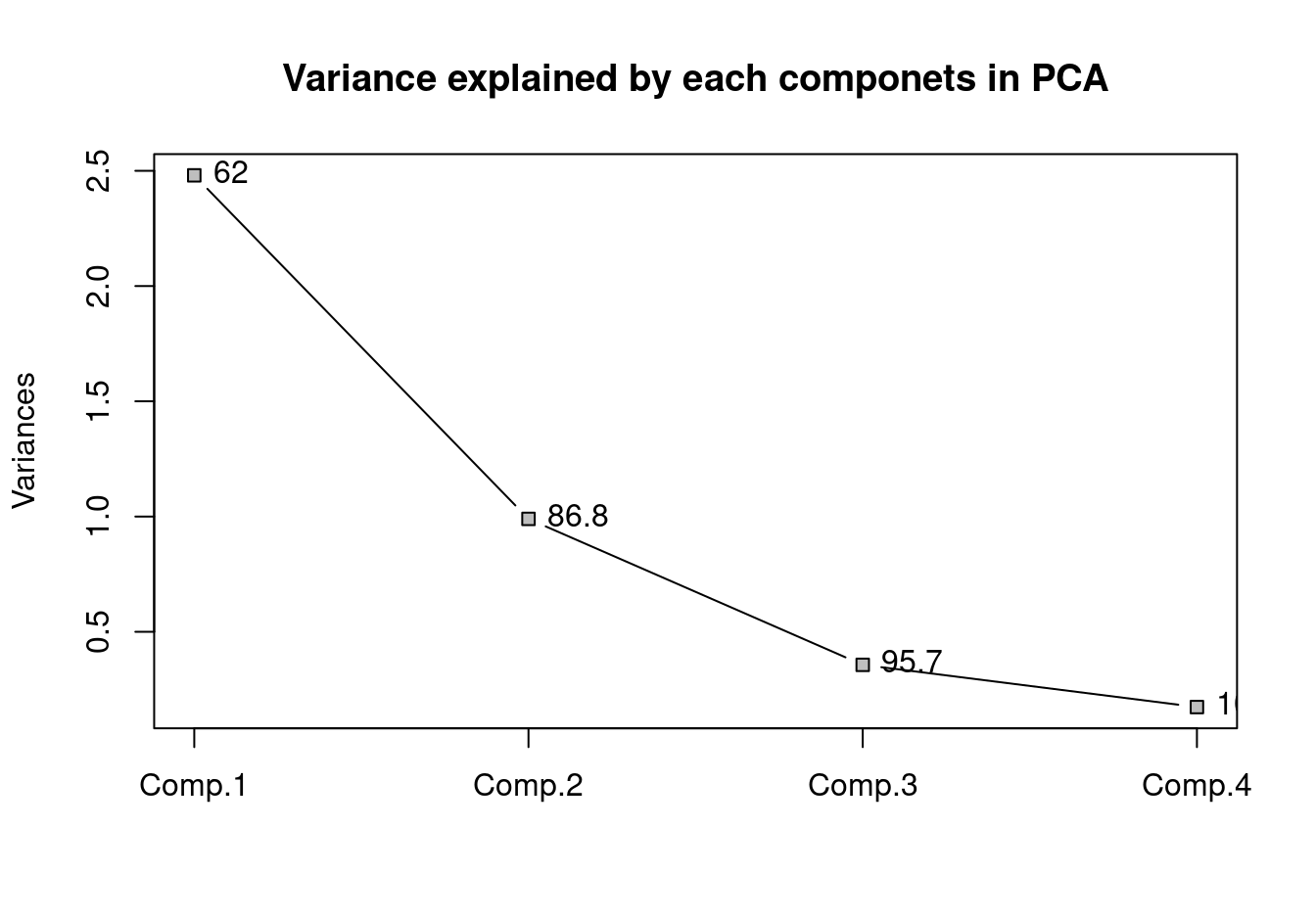# Interpreting Biplot

statistics
Author

therimalaya

Published

March 18, 2017

Obviously, the name suggests itself that there are two aspect of data plotted in latent scale. Biplot in case of principal component analysis (PCA) plot both variable and observation in latent scale (scores and loadings). Throughout this article, I will use `USArrests` data from `datasets` package. Lets fit a PCA model using `princomp` function. However, this can also be done using `prcomp`. The later one uses singular value decomposition while the former one uses eigenvalue decomposition. Here I have used correlation rather than covariance to compute principal components because there are large values in case of `Murder` and `UrbanPop`

``````pc.a <- princomp(USArrests, cor = TRUE)
plot(pc.a, type = "lines", pch = 22, bg = "grey",
main = "Variance explained by each componets in PCA")
text(1:4, pc.a\$sdev^2, pos = 4,
labels = round(cumsum(pls::explvar(pc.a)), 1))
box()``````Variance of each components and the proportaion of the total variance explained for each additional components is displayed as label

Here, with three components, we have captured 95.7 percent of total variation present in `USArrests` dataset. Lets get the biplot and try to understand it.

``````library(ggfortify)
autoplot(
pc.a, label = T, shape = FALSE,